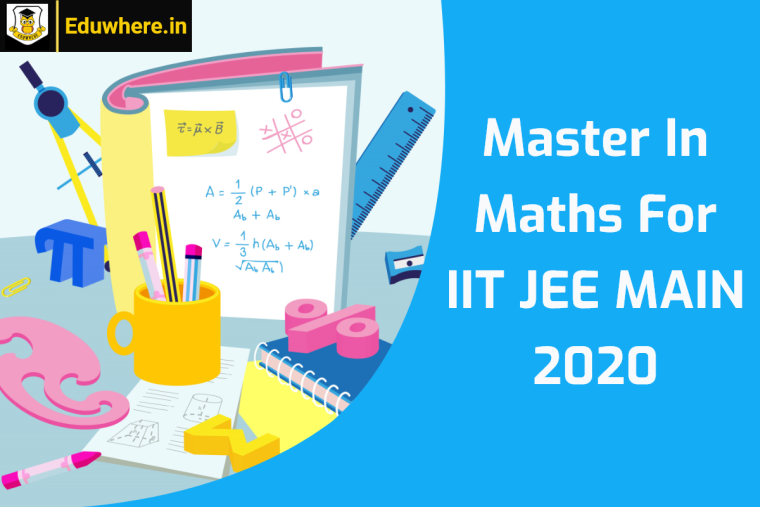## IIT JEE Main 2020: Tips to master in Maths sectionIIT JEE 2020 examination will be conducted in 2 sessions in the month of January and April for the candidates. When it comes to Maths section of IIT JEE examination, practice is the only key to success. Considered as tricky as well as a scoring part of the examination, Mathematics requires regular practice no matter whether you are going for coaching or preparing on your own.

One should always focus on being thorough with the important formulae for Maths and believe in various shortcuts and important formulae for IIT JEE exams.

Click here to take the online mock test for IIT JEE at Eduwhere.in

Take a look at the IIT JEE Main Maths syllabus and important topics to be covered.

 Sets, Relation and Function Complex Numbers and Quadratic equation Matrices and Determinants Mathematical Induction Permutations and Combinations Binomial Theorem Sequences & Series Limit, Continuity and Differentiability Integral Calculus Differential Equations Three-Dimensional Geometry Trigonometry, Vector Algebra Coordinate Geometry Mathematical Reasoning Statistics and Probability

Best Books for IIT JEE Main 2020 Maths preparation

• You will come across plenty of study material in the market for your exam preparation. However, here is a list of all the top books that can be referred for the IIT JEE Main 2020 examination.
• Coordinate Geometry by S.L. Loney

• R.D. Sharma for Class XI & XII

• Vishal Joshi ( advance level problems in mathematics)

• Trigonometry by S.L. Loney

• Cengage series by G.Tewami

• Higher Algebra by Hall Knight

• Problems in Calculus of One Variable by L.A. Maron

• NCERT textbook of class 11th and 12th

• Play with graphs by Amit Agarwal

Most Important Topics: Matrices & determinants, Integrals, 3-D geometry, Vector algebra, Probability, Conic section, Trignometry, Sets relation & function

Important Topics: Differential equation, Limits, Quadratic equation, Circle, Binomial theorem, Sequence & Series, Continuity & derivability, Permutation & combination

Good to know Topics: Mathematical reasoning, Height and distance, Application of derivative, Mathematical induction, Application of integrals, Inverse Trignometry

The first session of IIT JEE 2020 will be conducted in January. Candidates don’t have much time left for the same along with board exams in parallel. Considering that Mathematics can be a scoring topic, one should start preparing like an early bird. The earlier you start, the better you get every single day.

• Prepare a chart for important formulae: It is essential to know all the formulae of Maths to solve questions correctly. For topics like Trigonometry, Probability and Geometry, you won’t be able to score without being well-versed with the important formulae.

• Previous year question papers: Preparing for a subject and solving questions from the previous year papers can be helpful in self-analysis. Try to solve 1-2 question papers every week while the IIT JEE Main 2020 preparation phase.

• Focus on time: Getting correct answers is essential but not at the stake of too much time. While you practice from various question papers, ensure that you don’t spend more than expected time over a single question. Try giving special attention to topics that eat your time and note time while practising it.

• Work hard on Calculus and Algebra: Calculus and Algebra are considered as the most important topics which you just can’t afford to miss. Be thorough with both the topics and prepare wisely such that you are able to solve all the questions within time.

• Take online mock tests: Preparing with online mock test platforms like Eduwhere.in can help in having a complete exam like environment. Here you can get the detailed analysis, complete results and solution of the mock test.

Good Luck with your upcoming examination!

1

1458